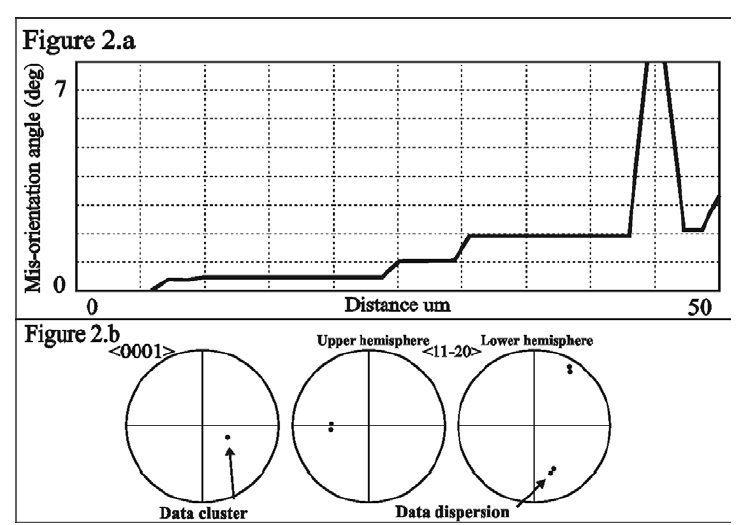Figure 2.a – This is a mis-orientation profile graph corresponding to transect line 1 on Figure 1. It shows the changing mis-orientation angle from the start point (detrital grain) to the outgrowth, which in this instance is 7°. Therefore the detrital grain and the outgrowth are not in crystallographic continuity.
Figure 2.b – This is a set of pole figures plotted on stereonets. It shows data for 6 data points picked from the detrital grain and the outgrowth area (from transect line 1 in Figure 1) in order to represent the misorientation as a rotation. The pole figures for <11-20> (a-axes) show a dispersion of data. The pole figure for the <0001> (c-axis) shows a cluster of data and therefore that a rotation axis for the misorientation rotations represented by transect line 1 is parallel to the direction of this cluster, i.e. parallel to the c-axis. This would indicate that there is a rational crystallographic rotation, i.e. that final growth stages were controlled and not random. (N.B. a range of rotation axes has been found, not only those that are parallel to the c-axes.)